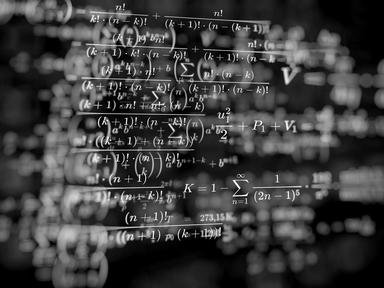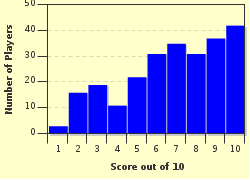FREE! Click here to Join FunTrivia. Thousands of games, quizzes, and lots more!# Calculus Fun (or My Head Hurts!) Quiz

### This quiz is based on infinitesimal calculus (specifically single-variable). It involves the history, definitions, and some problems to solve. Good luck!

A multiple-choice quiz by hausc018. Estimated time: 4 mins.

Author
hausc018
Time
4 mins
Type
Multiple Choice
Quiz #
335,741
Updated
Dec 03 21
# Qns
10
Difficulty
Average
Avg Score
7 / 10
Plays
736
Last 3 plays: Guest 204 (7/10), Guest 112 (3/10), Guest 176 (2/10).
This quiz has 2 formats: you can play it as a or as shown below.
Scroll down to the bottom for the answer key.
1. What is the broadest definition of calculus?
Hint

the study of collections of data
the study of operations and applications
the study of change
the study of shape

#### NEXT>

2. What are the two main branches of calculus?

Hint

probability and trigonometry
differential and probability
differential and integral
integral and probability

#### NEXT>

3. Who is widely considered to be the father of calculus (or the father of differential and integral calculus)? Hint

Both
Gottfried Wilhelm Leibniz
Sir Isaac Newton
Neither

#### NEXT>

4. When differentiating a function, what does the answer mean? In other words, what does the derivative of a function represent? Hint

neither
the rate of change of a function at a specific value
both
the slope of the tangent line to a curve

#### NEXT>

5. When integrating a function, what does the answer mean? In other words, what does the integral of a function represent? Hint

the area under the graph of a funtion bounded by a closed interval [a,b]
the slope of the tangent line to a curve
the rate of change of a function at a specific value
the time it takes to reach the limit of a function

#### NEXT>

6. What does it mean to find the limit of a function? Hint

the area under a curve between two points
the rate of change between two functions
the behavior of a function as it approaches a specific input
the slope of the tangent line to a cuve

#### NEXT>

7. Consider 'x' to be a variable. What is the derivative of 12x^4 + 10x^3 - 5x^2 + 16 with respect to x? Hint

48x^4 + 30x^3 - 10x^2
48x^3 + 30x^2 - 10x
48x^3 + 30x^2 - 10x + 16
12x^3 + 10x^2 - 5x

#### NEXT>

8. Consider 'x' to be a variable. What is the integral of 18x^2 - 10x + 3 with respect to x? Pick the most correct solution. Hint

9x^2 - 10x + 3
6x^3 - 5x^2 + 3x + C
6x^3 - 5x^2 + 3x
9x^2 - 5x + 3+ C

#### NEXT>

9. Suppose the integral was already performed on an equation. What is the simplified answer if you now take the integral from 0 to 1 for the following integrated equation:

8x^3 + 3x^2 - 4x + C

(Hint: closed interval [a=0, b=1])
Hint

7 + C
11 - C
11
7

#### NEXT>

10. Which of the following is not a known series used in calculus? Hint

Geometric
Taylor series
Harmonic
Simpson's Rule

 (Optional) Create a Free FunTrivia ID to save the points you are about to earn:Select a User ID:Choose a Password:Your Email:

Quiz Answer Key and Fun Facts
1. What is the broadest definition of calculus?

Calculus involves many other components when defining it, but in general it is the study of change. The study of operations and applications is algebra. The study of shape is geometry. The study of collections of data is statistics.
2. What are the two main branches of calculus?

The Fundamental Theorem of Calculus relates the two branches by stating they are inverse operations of each other.
3. Who is widely considered to be the father of calculus (or the father of differential and integral calculus)?

Today, they both share credit for developing differential and integral calculus. It has long been disputed as to who actually developed it first. Leibniz developed infinitesimal calculus independently and published his work first, but Newton began work on it before and took many years to publish his work. Leibniz focused more on systematic ways to solve problems and came up with appropriate symbols while Newton came up with rules, series, and functions in general.
4. When differentiating a function, what does the answer mean? In other words, what does the derivative of a function represent?

The answer is both because there are two ways in defining the derivative of a function: physically and geometrically. The most common forms of differential notation are Leibniz notation, which is noted as dy/dx and the Legrange notation or prime notation, which is noted as f'(x). Both notations are defined as the derivative of y with respect to x.
5. When integrating a function, what does the answer mean? In other words, what does the integral of a function represent?

Answer: the area under the graph of a funtion bounded by a closed interval [a,b]

Essentially, an integral is the anti-derivative. It is solved by taking the anti-derivative of the function with respect to x from the closed interval 'b' and subtracting the anti-derivative of the function with respect to x from the closed interval 'a'.
6. What does it mean to find the limit of a function?

Answer: the behavior of a function as it approaches a specific input

When determining the limit of a function, you are seeing what value (if any) the function is as it approaches a specific input. The limit may approach a constant, but it may also approach infinity or zero. Depending on the function, it may not even exist in either case.
7. Consider 'x' to be a variable. What is the derivative of 12x^4 + 10x^3 - 5x^2 + 16 with respect to x?

Answer: 48x^3 + 30x^2 - 10x

The formula for finding a derivative of a single-variable equation is:

f'(x) = (y*C)x^y-1, where C is a constant

Therefore, the answer is obtained by applying the formula in this way:

(4*12)x^4-1 + (3*10)x^3-1 - (5*2)x^2-1 + (0*16)

= 48x^3 + 30x^2 - 10x
8. Consider 'x' to be a variable. What is the integral of 18x^2 - 10x + 3 with respect to x? Pick the most correct solution.

Answer: 6x^3 - 5x^2 + 3x + C

The formula for finding an integral of a single-variable equation is:

F(x) = (Bx^y+1)/(y+1) + C, where B, C are constants

Therefore, the answer is obtained by applying the formula in this way:

(18x^2+1)/(2+1) - (10x^1+1)/(1+1) + (3x^0+1)/(0+1) + C

= (18x^3)/3 - (10x^2)/2 + (3x)/1 + C

= 6x^3 - 5x^2 + 3x + C
9. Suppose the integral was already performed on an equation. What is the simplified answer if you now take the integral from 0 to 1 for the following integrated equation: 8x^3 + 3x^2 - 4x + C (Hint: closed interval [a=0, b=1])

To simplify the integral from 0 to 1, use this formula:

F(x) = F(b) - F(a), where a and b are the bounds (in this case, a=0 and b=1)

Therefore, the answer is obtained by applying the formula in this way:

[8x^3 + 3x^2 - 4x + C]0-->1

= [8(1) + 3(1) - 4(1) + C] - [8(0) + 3(0) - 4(0) + C]

= [8 + 3 - 4 + C] - [0 + 0 - 0 + C]

= [7 + C] - [C]

= 7
10. Which of the following is not a known series used in calculus?

A series is the sum of the terms in a sequence and can be definite or indefinite. Simpson's Rule is used to approximate definite integrals, not series.
Source: Author hausc018

This quiz was reviewed by FunTrivia editor crisw before going online.
Any errors found in FunTrivia content are routinely corrected through our feedback system.
Most Recent Scores
May 26 2023 : Guest 204: 7/10
May 19 2023 : Guest 112: 3/10
May 12 2023 : Guest 176: 2/10
May 12 2023 : TERRYHURST22: 8/10
May 11 2023 : Guest 109: 9/10
May 01 2023 : Guest 165: 6/10
Apr 14 2023 : Guest 98: 7/10

Score Distribution